International Journal of Economy, Energy and Environment
Volume 1, Issue 2, October 2016, Pages: 16-23

CFD Simulation Studies for Vertical Temperature Profile, Pitch Optimisation and Parametric Study of Borehole Heat Exchanger

Shiv Lal1, *, Subhash Chand Kaushik2, Pradeep Kumar Bhargava3

1Department of Mechanical Engineering, Rajasthan Technical University Kota, India

2Central for Energy Studies, Indian Institute of Technology, Delhi, India

3Central Building Research Institute, Roorkee, India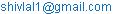(S. Lal)

*Corresponding author

Shiv Lal, Subhash Chand Kaushik, Pradeep Kumar Bhargava. CFD Simulation Studies for Vertical Temperature Profile, Pitch Optimisation and Parametric Study of Borehole Heat Exchanger.International Journal of Economy, Energy and Environment. Vol. 1, No. 2, 2016, pp. 16-23. doi: 10.11648/j.ijeee.20160102.11

Received: July 23, 2016; Accepted: August 10, 2016; Published: September 6, 2016

Abstract: In this communication, simulation studies of a borehole heat exchanger are worked out through computational fluid dynamics (CFD) software. A two dimensional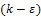realizable turbulent model with standard wall function is used to evaluate the temperature variation along with depth of BHE, pitch optimization and to determine the effect of two dimensionless parameters as ratio of pitch to borehole diameter and ratio of borehole to pipe diameter. The predicted results are validated through experimental data; and statistical assessment shows a good agreement between simulated and experimental results. The tube air temperature is proportional to depth in cooling mode and BHE can decrease the temperature of air by 13-14°C when ambient temperature observed by 41°C. The optimised pitch for 8 inch borehole and 2 inch diameter U-tube is found to be 4 inch, however two U-tubes are recommended for enhanced performance. The effective borehole to tube diameter ratio is estimated by 4. The BHE system can be used for heating and cooling of buildings it is a feasible solution for sustainable development.

Keywords: Borehole Heat Exchanger, Computational Fluid Dynamics, Optimization

Contents

1. Introduction

The solar and ground source passive space conditioning methods are gaining an increasing popularity in the residential and commercial buildings. It will contribute to reduction of the global energy consumption. The ground temperature below 4 m or more depth remains constant and it is equal to the annual mean ambient temperature . The earth air tunnel heat exchanger is one of the general applications of ground heat resources. The earth air tunnel may be classified into two categories as horizontal and vertical, and the vertical tube heat exchanger is just working a borehole heat exchanger. Kaushik et al. , experimentally analysed the horizontal earth air tunnel heat exchanger and reported the cooling / heating potential for Indian climatic condition. A Borehole heat exchanger (BHE) uses earth as a heat source and sinks to extract or reject the thermal energy from/into the ground. It is one of the examples of the geo-exchange and the hot/cold air passing through the BHE and producing air can be used in buildings space conditioning. The utilization of ground source as borehole heat exchanger (BHE) system is environment friendly, causing fewer emissions than the conventional energy sources. In heat transfer analysis of BHE system one need to understand the dependence of various parameters like geometrical configuration of the pipes, thermal and fluid properties of the heat transfer mediums surrounding system.

Sanner B.  studied the shallow geothermal energy wells and observed that the temperature of the ground is reduced to steady temperature (Average constant temperature throughout the year) at 10 to 20 m depth and further the earth temperature will be increasing on average 3°C for each 100m depth. Zeng et al.  developed a quasi-three dimensional model for heat transfer analysis of BHE and traced a fluid temperature profiles along the borehole depth. It was also found that the double U-tube boreholes have better performance than single U-tube borehole with reduction in borehole resistance of 30-90%.

Sagia et al.  evaluated the borehole thermal resistance. It was also found that the borehole thermal resistance is a function of borehole to tube diameter ratio, shank spacing to borehole diameter ratio and thermal conductivity of the grout material. The effect of conduction in borehole was examined by finite element analysis (FEA) through COMSOL (A heat transfer module) multi-physics, for which three different configuration pipes have been used.

Beier et al.  developed a model for predicting the thermal response test (TRT) and "Sandbox" laboratory test for a U-tube borehole heat exchanger. Whereas 12.6 cm diameter borehole in which aluminium pipe was used with 3.34 cm diameter U-tube of 18.3 m length. The system was used as ground heat exchanger (GHE) and found that borehole resistance is increased with increase in soil thermal conductivity. A three dimensional heat transfer model have been developed by Rees and He  to evaluate the heat transfer and fluid flow performance of BHE. The developed model have been validated through experimental and analytical results, and it can used to calculated the transient heat transfer rates over both long and short time scale. Sharqawy et al.  developed a mathematical model for thermal resistance of BHE and simulated in CFD for different borehole geometries and compared their results with results of Gu and O Neal , Remund  and Shonder and Beck  models. Gustafsson and Westerlund  also simulated the thermal resistance in a U-tube BHE through CFD technique. Bouhacina et al.  analysed the water in tube BHE for turbulent fluid flow and k-epsilon model was used to simulate the developed algorithm. A modified multilayer model for borehole ground heat exchangers with an inhomogeneous ground water flow have been developed by Lee and Lam .

This technology is not matured till now and research in this field is needed to simulate the single and multi U-tube BHE models. So it can be publicised as best option of energy conservation. In this paper, the CFD simulation of BHE has been presented for the temperature variation in the U-tube. The pitch and diameters are also optimised and thermal resistance of experimental BHE have also been evaluated.

2. Material and Methods

2.1. Governing Equations

The actual heat is transferred through the conductive and convective mode in a single U-tube borehole heat exchanger. Whereas the first convective heat transfer takes place between water and U-tube material, secondly conductive heat transfer in the tube wall and finally convective heat transfer between tube material and air in the tube. The following assumptions have to be made for CFD analysis of BHE in this study.

1.  There is no thermal contact resistance at any interface.

2.  The initial temperature is uniform throughout the whole domain.

3.  The grout material as water is homogeneous.

A two dimensional model is adapted for fluid flow and heat transfer analysis in CFD to ease the computational effort. The governing equations associated with water, soil, air and tube components. There is no internal heat generation in the component’s, and pressure inside the tube is equal to the atmospheric pressure are assumed for the solution. The general energy equation is defined by FLUENT software  for solid (soil, tube) component as follows: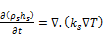(1)

Where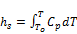(2)

Water is an incompressible fluid, and the governing equations are based on the continuity and energy conservation equations. These equations are as follows:

Continuity:(3)

Navier-Stokes equation (RANS):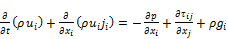(4)

Energy equations: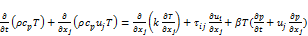(5)

Equation for the turbulent kinetic energy (k):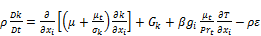(6)

Equation for the energy dissipation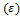: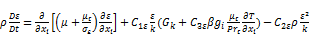(7)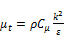(8)

And coefficient of thermal expansion,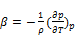(9)

The above model constants for the turbulent model can be found in Fluent (6.3.26). The default value of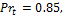and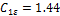,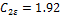,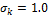,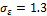,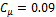, and the fully developed geometry is used to reduce the complexity of the problem.

2.2. Computational Effort

A Work station (Dell precision T7400 of 800MHz multicore Intel Xeon processors, 64GB RAM, 1TB HDD) is used for the simulation study, which needs less amount of computational time for one steady-state solution.

2.3. Boundary Conditions

A static pressure boundary condition is used as there is no pressure difference at BHE exit which means pressure at inlet and outlet of BHE are equal to atmospheric pressure. The inlet temperature of BHE used in simulation study for a particular time has been taken as the ambient temperature for the same time. The whole system is divided into three zones as tube under water, tube above water (submerged in air) and air. The static temperature of water-tube surface is assumed as 25°C, and noted that the air-tube static temperature is found two degree higher than the water-tube temperature. The steel is selected for U-tube. The boundary types were set as follows:

a)  Inlet was set as a velocity inlet

b)  Exit was set as outlet

c)  Tube wall (side wall) was set as a wall into two parts as air-tube wall (one-third from top) and water-tube wall (two-third part of total length of borehole) at constant temperatures.

2.4. Important Input Parameters

A 2-Dimensional steady, Realizable k-epsilon turbulent model with standard wall function was used for the study. Some of the important properties of materials and modeling parameters required for the CFD simulation of BHE are shown in table given as below.

Table 1. Input parameters for CFD study.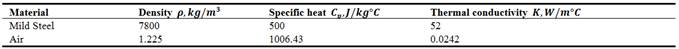2.5. Numerical Simulation

The numeric simulation is based on step by step method which is used to develop geometry, grid generation, applying boundary conditions, and ultimately simulates it in FLUENT up to convergence.

2.6. Geometry and Meshing

A BHE consists of a vertical borehole with a U-tube heat exchanger. A borehole of 203.2mm diameter and18.29m depth is drilled near the symmetric house for experimental studies at CSIR-CBRI, Roorkee, India. A PVC pipe perforated upto 6.1m from bottom is installed for full depth in the borehole. A Galvanised Iron (G.I.) pipe of 50.8mm diameter is used as U-tube as shown in Figure 1. A two dimensional geometry based on designed dimensions is developed in workbench design modular software. The grid was generated using Meshing software which compatible to FLUENT [ANSYS workbench 14.0].

The quality of the grid plays a direct role on the quality of the analysis, regardless of the flow solver used. The grid can be shaped to be body fitted through stretching and twisting of the block. Mesh quality is the key for convergence and accurate CFD solutions in case of complex geometry especially for viscous flows. The medium mesh of specific sizing has been generated accordingly to the requirement and again mesh (grid) has been double refined near the edges. After that it was checked and optimised. After meshing the model is updated to next level i.e. FLUENT. In this process problem (.msh) model file is setup as pressure based, steady, and 2 D spaces planer. The energy, viscous model have been used for the simulation and k-epsilon realizable turbulent model with standard wall function also being used. And next operations are as defining the materials and check for the particular zone, correct the cell zone conditions as solid, and liquid, putting all the boundary conditions for each defined zones, initialize the solution for standard initialization start from inlet and finally run the calculations upto converging the solution.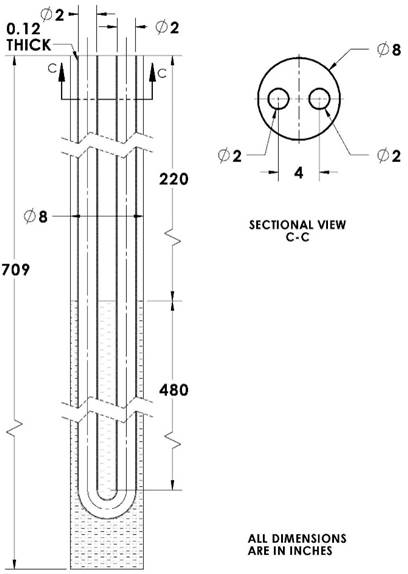Figure 1.Borehole heat exchanger model for CFD simulation.

3. Results and Discussion

CFD model of single U-tube BHE as shown in Figure 1 is used to simulate for its thermal performance. The inlet velocity and temperature is set for 18.9 m/s and 40°C respectively. The far field boundary temperature was set at 25°C. The calculated heat transfer coefficient was 10.2-13.3 W/m2, it is higher from heat transfer coefficient of ground coupled heat pump (GCHP, h=1.56 W/m2) due to higher air velocity inside the tube and U-tube is dipped in ground water.

3.1. Model Validation

Two dimensional CFD model of BHE have been simulated under the experimental conditions, where experimental data also collected in the month of May 2013. The experimental set-up which is used for the study is situated at CSIR-CBRI Roorkee, India. The CFD and experimental output air temperature are graphical presented in Figure 2. It is seen that, there is small variation between the modelling and experimental results and found between 1.08 to 2.48°C. The total MBE and RMSE are evaluated by 1.74 and 1.79 respectively. The pressure is reduced from inlet to outlet that’s why the velocity of the air is higher at exit than inlet of BHE. The pressure drop is reduced mainly due to pipe friction. The CFD and experimental results of pressure drop is shown in Figure 6, it is noted that the pressure drop error observed by 14-44Pa, where MBE and RMSE are evaluated by 25Pa and 26.98Pa respectively. A good agreement observed between CFD and experimental results.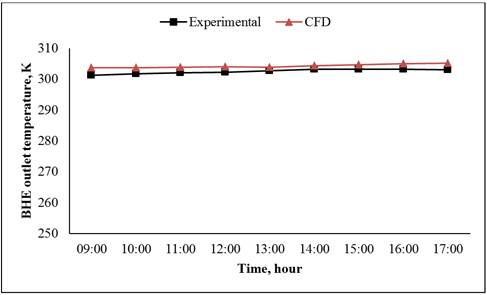Figure 2. Validation of Simulated output temperatures to the experimental.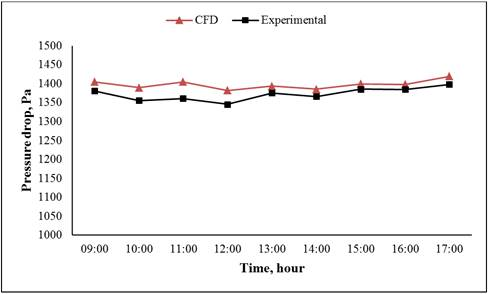Figure 3. Pressure drop between inlet and outlet of BHE for a specific day.

3.2. Temperature Variation with Depth of BHE

The temperature contour of single U-tube BHE is shown in Figure 4, it is seen that the air temperature is reduced in left limb from top to bottom but in right limb it is likely to be constant the similar results are graphically presented in Figure 5, whereas Line 17 is represented the left limb and line 18 represented the right limb. Approximately two-third part of the BHE is dipped in to water and 1/3 part is open to borehole air. From the experimental analysis, outlet air temperature is found by1-3°C higher than the ground water temperature. The similar trends are followed by CFD results as shown in Figure 5. The ambient temperature is observed by 41°C and the air temperature is reduced by approx. 13-14°C after flow through BHE. The cooling effect is proportionally varying to the ambient temperature (above 25°C) because groundwater temperature is remains constant at 25°C in the Roorkee, India. This ground water temperature is approximately equal to the average annual solair temperature. It is also observed by experimental for a year of 2012.Figure 4. Temperature contour of single U-tube BHE.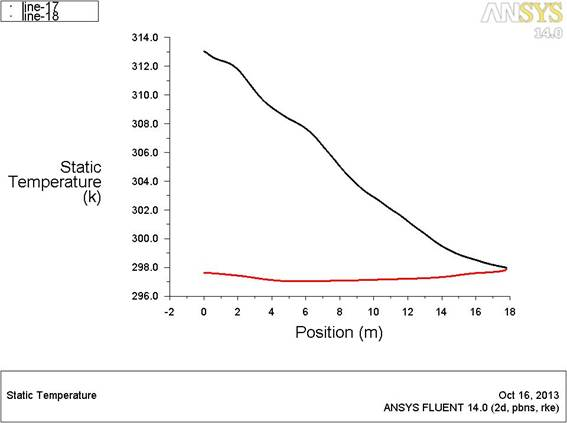Figure 5. Vertical temperature variation in U-tube.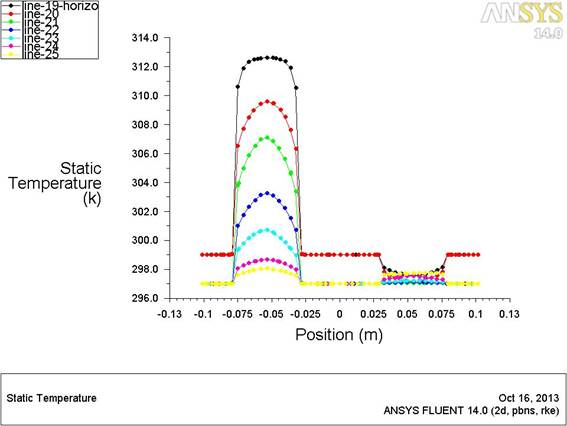Figure 6. Temperature profile at various cross sections in single U-tube BHE.

Figure 6 shown the temperature profile at various horizontal positions from top to bottom, the temperature profile in the tube is likely to be parabolic. The profile in the U-tube is exposed that the temperature at centre of tube is higher than the sides and the lowest temperature of air found at closure to the tube surface.

3.3. Pitch Optimization in Single U-tube BHE

Sharqawy et al.  given the best fit conditions for design of a BHE, where it proposed two ratios one is called pitch to borehole diameter (PD) and diameter ratio (Dr=Borehole diameter/U-tube diameter).The specified range of (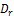) and (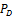) is recommended by: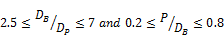(10)

The proposed equation for calculating the thermal resistance of BHE is given by: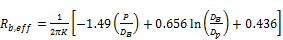(11)

In our experimental study these dimensionless values are examined as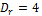and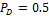respectively. So the effective pipe to borehole thermal resistance found to be 0.1667m.K/W. Where grout material is water and its thermal conductivity is 0.58 W/mK.

The PD is one of the dimensionless parameter which used to optimise the pitch based on thermal resistance. The CFD model is operated for three different PD (0.356, 0.5 and 0.625) as shown in Figure7. From the temperature contour, it is shown that a minimum temperature at exit is found for PD=0.5 and maximum for PD=0.375 for single U-tube. So that the optimum pitch value is 4 inch for 8 inch borehole diameter (based on PD=0.5) and 2 inch single U-tube diameter.

When pitch is optimised it is found that the performance of BHE is defined by the effective heating/cooling rate on highest air flow rate. Jalaluddin et al.  verified that the two U-tube BHE gives higher performance than the single U-tube. From Figure 7(b), it is seen that the outlet temperature is slightly increasing but from two tubes it gives higher volumetric effective air for cooling mode in summer, and similar result can be evaluated for heating in winter.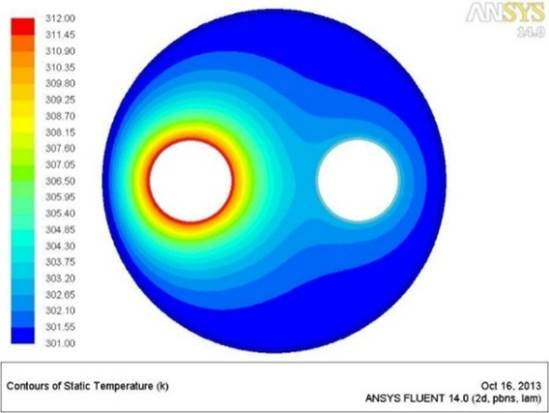(a). p/dB=0.5, single U-tube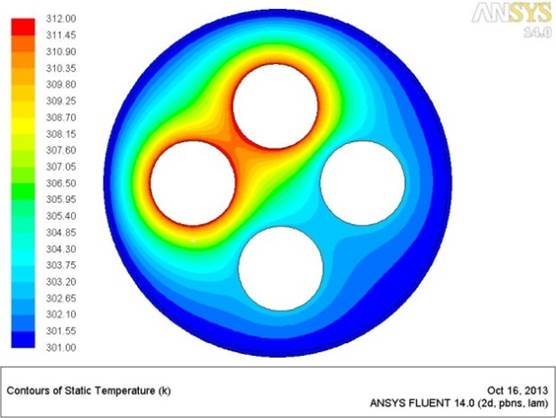(b).p/dB=0.5, double U-tube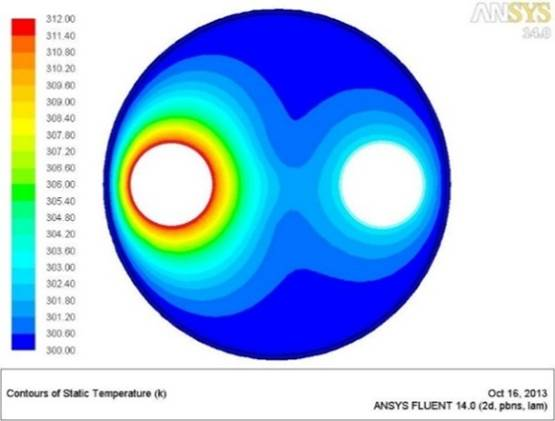(c). p/dB=0.625, single U-tube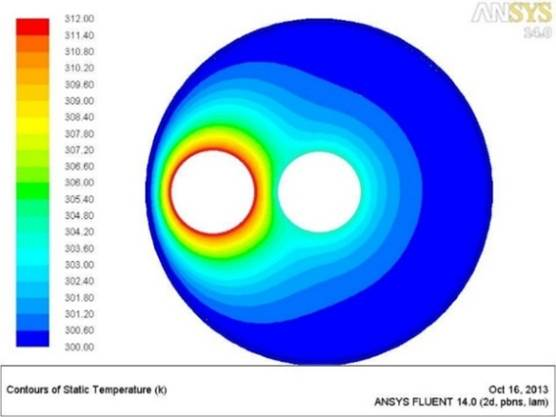(d).p/dB=0.375, single U-tube

Figure 7. Distribution of temperatures (in °C) within boreholes of (PD) different geometries.

3.4. Effect of Ratio of Borehole to Pipe Diameter (Dr)

The CFD model is simulated for four different Dr (2.67, 3.2, 4 and 8). The temperature contour for different Dr is shown in Figure 8. It is shown that the temperature contour is shifted towards the borehole pipe when the Dr is increases. The most suitable Dr is 4 for double U-tube BHE and Dr=8 is not feasible according to Sharqawy et al. , the feasible condition for Dr is (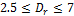). It is found that the Figure 8(b) configuration is most feasible solution for single or double U-tube BHE. It is also noted that the effective borehole resistance is increasing with increasing Dr.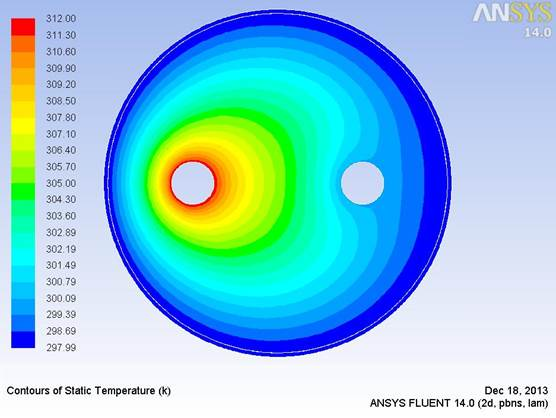(a). Dr=8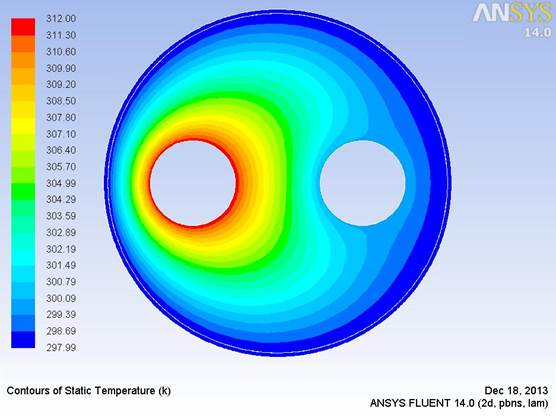(b). Dr=4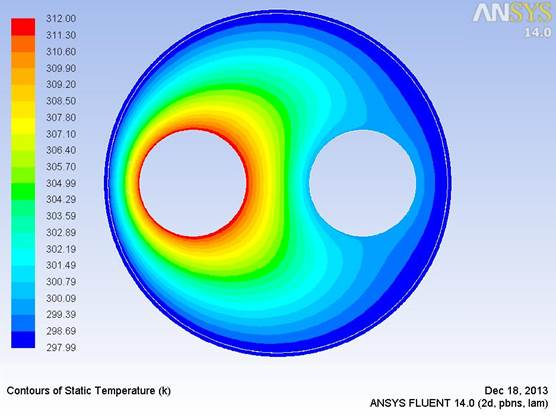(c). Dr=3.2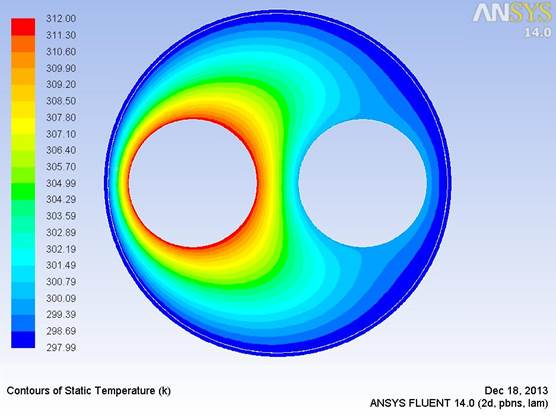(d). Dr=2.67

Figure 8. Distribution of temperatures (in °C) within boreholes of different (Dr) geometries.

4. Conclusions

Two dimensional CFD model has been developed and validated through the experimental data. The MBE and RMSE are evaluated by 1.74 and 1.79, and found a good agreement between the simulated and experimental results. The maximum temperature of air is reduced in left limb (intake limb) of BHE; and air temperature slightly rose in right limb (outlet limb). It is observed that the heat transfer is proportionally depends on the depth of BHE and the depth is optimised by the grout temperature and the economic analysis of the system. The maximum potential of heating and cooling is depends on the temperature difference between the extreme environmental temperature and the average annual solair temperature. It is most feasible solution for hot regions like Rajasthan, India and ice-cold regions like Himalayan region in India. It can be reduced the probability of freezing, so it has an important role for human comfort in cold regions.

The temperature profile is found to be parabolic and it is well known pattern. The pitch has been optimised and estimated by 4inch (101.6 mm). The thermal resistance is depends on the Dr and PD, both the parameters are evaluated and presented for different configurations. The single U-tube BHE gives significant performance but for higher performance author have suggested two U-tubes BHE.

Nomenclature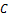Constant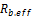Effective resistance of BHESpecific heat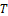Temperature, °CBorehole to U-tube diameter ratio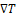Temperature difference, °C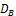Borehole diameterTurbulence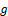Gravitational constant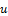Velocity, m/s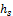Heat transfer coefficient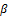Coefficient of thermal expansion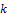Kinetic energyEnergy dissipation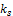Thermal conductivity of soil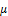Dynamic viscosity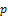Pressure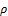Density of air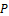PitchDensity of soilPitch to Borehole diameter ratio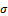Compressive stress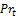Turbulent Prandtl numberShear stress

Acknowledgement

The author (Shiv Lal) gratefully acknowledges University College of Engineering, Rajasthan Technical University, Kota, Rajasthan (India) and IIT Delhi (India), for sponsorship under quality improvement program of government of India.

References

1. Kaushik,S.C.; Lal,S.; Bhargava P.K.(2013); Earth–air tunnel heat exchanger for building space conditioning: a critical review. Nanomaterials and energy; vol. 2(4), pp. 216-227, DOI: 10.1680/nme.13.00007.
2. Kaushik, S.C.; Garg, T.; Lal, S. (2014); Thermal performance prediction and energy conservation potential of earth air tunnel heat exchanger for thermal comfort in India. Journal of renewable and sustainable energy, 6, pp. 013107 (1-12), DOI: 10.1063/1.4861782.
3. Sanner,B. (2001); Shallow geothermal energy; GHC Bulletin, pp.19-25.
4. Zeng,H.; Diao,N.; Fang, Z. (2003); Heat transfer analysis of borehole on vertical ground heat exchangers. International journal of heat and mass transfer;vol. 46, pp. 4467-4481, DOI: 10.1016/S0017-9310(03)00270-9.
5. Sagia, Z.; Stegou, A.; Rakopoulos, C. (2012); Borehole resistance and heat conduction around vertical ground heat exchanger; The open chemical engineering journal, vol. 6, pp. 32-40.
6. Beier, R.A.; Smith, M.D.; Spitler, J.D. (2011); Reference data sets for vertical borehole ground heat exchanger models and thermal response test analysis; Geothermics, vol. 40, pp. 79-85, DOI: 10.1016/j.geothermics.2010.12.007.
7. Rees,S.J. and He, M. A. (2013); three-dimensional numerical model of borehole heat exchanger heat transfer and ﬂuid ﬂow; Geothermics, vol. 46, pp. 1-13, DOI: 10.1016/j.geothermics.2012.10.004.
8. Sharqawy, M.H.;Mokheimer, E.M.;Badr, H.M. (2009);Effective pipe-to-borehole thermal resistance for vertical ground heat exchangers; Geothermics, vol. 38, pp. 271-277, DOI: 10.1016/j.geothermics.2009.02.001.
9. Gu, Y.; O’Neal D.L. (1998); Development of an equivalent diameter expression for vertical U-tubes used in ground-coupled heat pumps; ASHRAE Transactions,vol. 104, pp. 347–355.
10. Remund, C.P. (1999); Borehole thermal resistance: laboratory and field studies; ASHRAE Transactions,vol. 105, pp. 439–445.
11. Shonder, J.A.; Beck J.V. (1999); Field test of a new method for determining soil formation thermal conductivity and borehole resistance; ASHRAE Transactions,vol. 106, pp. 843–850.
12. Gustafsson, A.M.; Westerlund, L. (2010); Simulation of the thermal borehole resistance in groundwater filled borehole heat exchanger using CFD; International journal of energy and environment,vol. 1(3), pp. 399-410
13. Bouhacina, B.; Saim, R.;Benzenine, H.; Oztop, H.F. (2013). Analysis of thermal and dynamic comportment of a geothermal vertical U-tube heat exchanger. Energy and buildings, vol. 58, pp. 37-43, DOI: 10.1016/j.enbuild.2012.11.037.
14. Lee, C.K.; Lam, H.N. (2012); A modified multi-layer model for borehole ground heat exchangers with an inhomogeneous groundwater flow; Energy, vol. 47, pp. 378-387, DOI: 10.1016/j. energy.2012.09.056.
15. http://www.cfd-online.com/Wiki/Standard_k-epsilon_model, retrieved on December 10, 2013
16. Jalaluddin; Miyara, A.; Tsubaki, K.; Yoshida, F. (2011); Numerical simulation of vertical ground heat exchangers for ground source heat pumps;10 IEA heat pumps conference, pp.1-10

 Contents 1. 2. 2.1. 2.2. 2.3. 2.4. 2.5. 2.6. 3. 3.1. 3.2. 3.3. 3.4. 4.
Article ToolsAbstractPDF(3260K)